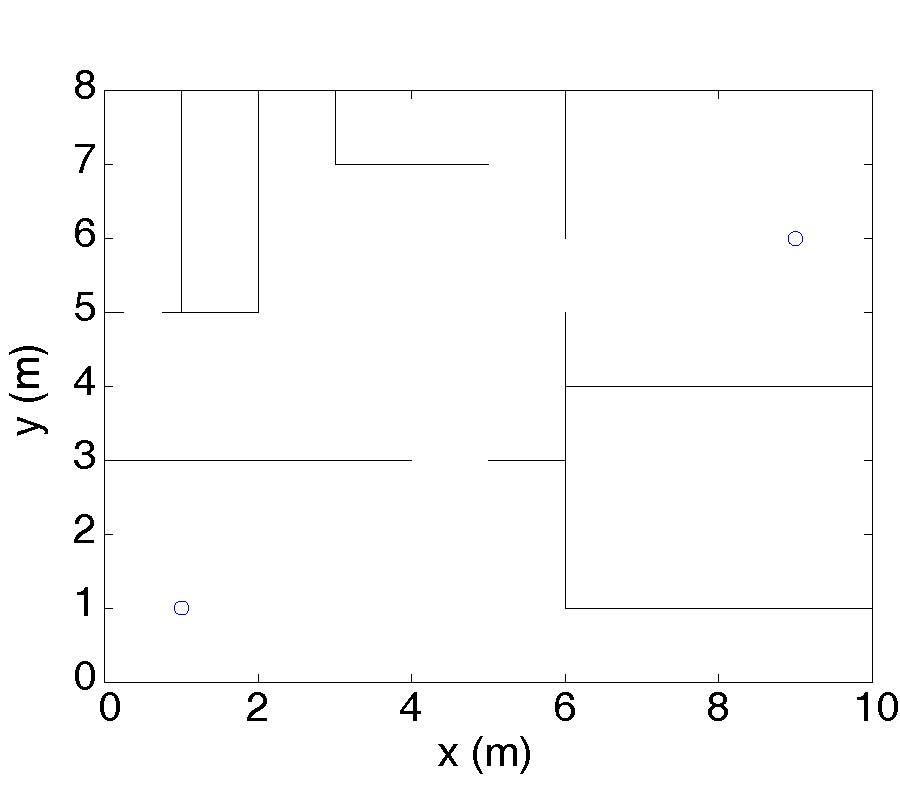# Vol. 21

Front:[PDF file] Back:[PDF file]
Latest Volume
All Volumes
All Issues
2011-10-19

#### Uncertainty Propagation and Sensitivity Analysis in Ray-Tracing Simulations

By Antoine Haarscher, Philippe De Doncker, and David Lautru
Progress In Electromagnetics Research M, Vol. 21, 149-161, 2011
doi:10.2528/PIERM11090103

## Abstract

Up to now, ray-tracing simulations are commonly used with a deterministic approach. Given the input parameters, the ray-tracing algorithm computes a value for the electric field. In this paper, we present a method that aims at computing the mean and standard deviation of the electric field. More precisely, we aim to obtain the probabilistic content of the electric field value and direction. We assume that this uncertainty results from input random variables which we consider uniformly distributed. Since ray-tracing computations have a high computational cost, we use spectral methods in order to optimize the number of simulations. We consider 2D electromagnetic propagation for the multi-path components, which can interact with the environment through four processes: transmission, single reflection, double reflection and diffraction. These are modelled using adequate coefficients. In order to calculate the polynomial chaos expansion coefficients, we use the projection method and Gauss-Legendre quadratures. These coefficients can then be used to determine the Sobol indices of input parameters. This is done in order to neglect variables in practical computation of the uncertainties.

## Citation

Antoine Haarscher, Philippe De Doncker, and David Lautru, "Uncertainty Propagation and Sensitivity Analysis in Ray-Tracing Simulations," Progress In Electromagnetics Research M, Vol. 21, 149-161, 2011.
doi:10.2528/PIERM11090103
http://www.jpier.org/PIERM/pier.php?paper=11090103

## References

1. ICNIRP, "International commission on nonionizing radiation protection guidelines for limiting exposure to time-varying electric, magnetic and electromagnetic fields (up to 300 GHz)," Health. Phys., Vol. 74, No. 4, 494-522, 1998.

2. Wiener, N., "The homogeneous chaos ," Am. J. Math., Vol. 60, 897-936, 1938.
doi:10.2307/2371268

3. Ghanem, R. G. and P. C. Spanos, "Stochastic Finite Elements-a Spectral Approach," Springer, Berlin, 1991.

4. Sudret, B., "Global sensitivity analysis using polynomial chaos expansion," Reliab. Eng. Syst. Safety, Vol. 10, No. 1016, 2007.

5. Le Maitre, O. P. , M. Reagan, H. N. Najm, R. G. Ghanem, and O. M. Knio, "A stochastic projection for method for fluid flow II --- Random process," J. Comput. Phys., Vol. 181, 9-44, 2002.
doi:10.1006/jcph.2002.7104

6. Sudret, B., "Uncertainty propagation and sensitive analysis in mechanical models and contribution to structural reliability and stochastic spectral methods," Universite Blaise Pascal, Clermont-Ferrand, 2007.

7. Sobol, I. M., "Sensitivity estimates for nonlinear mathematical models," Math. Modeling. Comput. Exp., Vol. 1, No. 14, 407, 1993.

8. Balanis, C. A., Advanced Engineering Electromagnetics, Wiley, New York, 1989.

9. Rohan, E., Introduction to Electromagnetic Wave Propagation, Artech, Boston, 1991.

10. Ida, N., "Engineering Electromagnetics," Springer-Verlag, 2004.

11. Luebbers, R., "Finite conductivity uniform GTD versus knife edge di®raction in prediction of propagation path loss," IEEE Trans. Antennas Propagat., Vol. 32, No. 1, 70-76, 1984.
doi:10.1109/TAP.1984.1143189